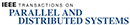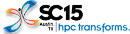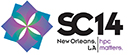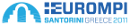Felix, qui, quod amat, defendere fortiter audet
Home -> Research -> Netgauge -> LogGPSHome
Publications
Awards
Research

NB Collectives
MPI Topologies
MPI Datatypes
Netgauge

LogGPS
OS Noise
eBB
Network Topologies
Ethernet BTL eth
ORCS
DFSSSP
Older Projects
cDAG
LogGOPSim
CoMPIler
Teaching
BLOG
Miscellaneous
Full CV [pdf]EventsPast EventsNetgauge - LogP, LogGP, and LogGPS Measurement

### Netgauge LogGPS (LogP, LogGP) Measurement Description:

The loggp pattern in Netgauge allows the precise measurement of LogP , LogGP , and LogGPS  parameters of MPI implementations. Only MPI is supported right now, but the required modifications to support different modules (e.g., TCP, UDP) should be minimal. The loggp pattern employs the techniques described in Low-Overhead LogGP Parameter Assessment for Modern Interconnection Networks .

#### General

The benchmarks use Netgauge's high-performance timers for different architectures. Users should make sure that the configure script detected the timer correctly and that it works reliably (no frequency scaling etc.). The benchmark results can be hard to interpret due to the used measurement method. We decided against returning single parameters because that could easily lead to wrong results or even negative parameters. Instead, the benchmark returns results for each message size (as it proceeds) and the quality of the fitting. A possible invocation would be:
```\$ mpirun -n 2 ./netgauge -s 1-8192 -x loggp
# Info:   (0): Netgauge v2.1 MPI enabled (P=2) (./netgauge -s 1-8192 -x loggp )
# initializing x86-64 timer (takes some seconds)
# Info:   (0): Warming module mpi up ... this may take a while
Testing 1 bytes 100 times:
L=0.6128  s=1  o_s=0.266  o_r=0.547  g=nan  G=nan (nan GiB/s) lsqu(g,G)=nan
Testing 1025 bytes 100 times:
L=0.6128  s=1025  o_s=0.458  o_r=1.163  g=0.448  G=0.000583 (13.405 GiB/s) lsqu(g,G)=inf
Testing 2049 bytes 100 times:
L=0.6128  s=2049  o_s=0.528  o_r=1.542  g=0.483  G=0.000478 (16.349 GiB/s) lsqu(g,G)=0.0877
Testing 3073 bytes 100 times:
L=0.6128  s=3073  o_s=0.622  o_r=1.806  g=0.534  G=0.000404 (19.338 GiB/s) lsqu(g,G)=0.1156
Testing 4097 bytes 100 times:
L=0.6128  s=4097  o_s=0.714  o_r=1.867  g=0.612  G=0.000328 (23.820 GiB/s) lsqu(g,G)=0.1706
Testing 5121 bytes 100 times:
L=0.6128  s=5121  o_s=0.781  o_r=2.050  g=0.689  G=0.000272 (28.745 GiB/s) lsqu(g,G)=0.2028
Testing 6145 bytes 100 times:
L=0.6128  s=6145  o_s=0.869  o_r=2.190  g=0.749  G=0.000236 (33.063 GiB/s) lsqu(g,G)=0.2127
Testing 7169 bytes 100 times:
L=0.6128  s=7169  o_s=0.967  o_r=2.326  g=0.801  G=0.000211 (37.018 GiB/s) lsqu(g,G)=0.2169
```
The actual parameters are reported for each data-size. The latency is half of roundtrip-time of a 1-byte message (and does not depend on the data-size). The send overhead is computed as described in  and the receive overhead is simply the time it takes to finish MPI_Recv() (and thus not very accurate). The parameter g and G are computed by the curve fitting. The curve fitting needs at least two points, thus, they can not be computed for the first measurement (nan). However, the more measurement points are considered, the more accurate are the results. The last parameter "lsqu(g,G)" is the least squares deviation of the fit for g,G. The lower this number is, the better the fit and the results. Please refer to  for details. Parameter changes are detected by sudden changes in the least squares deviation. Please refter to  for details. The benchmark also creates a file "ng.out" which can be plotted for visual analysis of the results. One possible plot in gnuplot would be: plot "ng.out" using 1:(\$4-\$3)/(\$2-1) . This plots the points that the g,G, line are fitted to. Or plot "ng.out" using 1:7 plots the send overhead for varying data sizes.

#### Getting the LogGPS parameters

Extracting the actual parameters from the output is difficult and requires some level of understanding of the used technique. Please refer to  in order to understand the measurement method. A rough guide for each of the parameters is given below:
• L: simply use the displayed L (round-trip/2). Sometimes it is advisable to substract o_s and/or o_r, however, this can lead to negative latencies (as o_s can happen after the message has been sent).
• o_s: is defined to be constant (per packet) in the LogP model, however, it is often not constant in practice (per message which might consist of multiple packets). You should use o_s of the desired packet size.
• o_r: is relatively imprecise and should be used carefully. Please contact the author if you know a precises measurement method for o_r.
• g: is approximately the point where the fitted curve crosses the y axis (s=0). However, some systems don't have ideal transmission curves. It is advisable to use a g with sufficiently many points to fit and a small lsqu(g,G).
• G: is the slope of the fitted g,G curve. It is advisable to use a g with sufficiently many points to fit and a small lsqu(g,G).
• S: is where the library switches from eager to rendezvous. While the library is not obliged to do this at all, it is commonly done on MPI libraries. The benchmark monitors the deviation and tries to detect protocol changes. However, it is safest to investigate the plot manually.

### References

 PMEO'07  T. Hoefler, A. Lichei and W. Rehm: Low-Overhead LogGP Parameter Assessment for Modern Interconnection Networks TU Chemnitz. In Proceedings of the 21st IEEE International Parallel & Distributed Processing Symposium, PMEO'07 Workshop, presented in Long Beach, CA, USA, IEEE Computer Society, ISBN: 1-4244-0909-8, Mar. 2007,David Culler, Richard Karp, David Patterson, Abhijit Sahay, Klaus Erik Schauser, Eunice Santos, Ramesh Subramonian, Thorsten von Eicken LogP: towards a realistic model of parallel computation ACM SIGPLAN Notices, Volume 28 , Issue 7 (July 1993), Pages: 1 - 12  Albert Alexandrov, Mihai F. Ionescu, Klaus E. Schauser, Chris Scheiman LogGP: Incorporating Long Messages into the LogP Model --- One step closer towards a realistic model for parallel computation Technical Report: TRCS95-09, University of California at Santa Barbara Santa Barbara, CA, USA  Fumihiko Ino, Noriyuki Fujimoto, Kenichi Hagihara LogGPS: a parallel computational model for synchronization analysis Proceedings of the eighth ACM SIGPLAN symposium on Principles and practices of parallel programming, Pages: 133 - 142 Year of Publication: 2001 ISBN:1-58113-346-4
 serving: 3.87.147.184:47310 © Torsten Hoefler